# Exercise E7.3 Triangles NCERT Solutions Class 9

## Chapter 7 Ex.7.3 Question 1

$$\Delta ABC$$ and $$\Delta DBC$$ are two isosceles

triangles on the same base $$BC$$ and vertices $$A$$ and $$D$$ are on the same side of $$BC$$ (see the given figure). If $$AD$$ is extended to intersect $$BC$$ at $$P$$, show that

(i) $$\Delta ABD = \Delta ACD$$

(ii) $$\Delta ABP = \Delta ACP$$

(iii) $$AP$$ bisects $$\angle A$$ as well as $$\angle D$$.

(iv) $$AP$$ is the perpendicular bisector of

$$BC$$.

### Solution

What is Known?

and are two isosceles triangles.

To prove:

i) $$\Delta ABD\cong \Delta ACD$$

ii) $$\Delta ABP\cong \Delta ACP$$

iii) $$AP$$ bisects $$\angle A$$ as well as $$\angle D$$.

iv) $$AP$$ is the perpendicular bisector of $$BC$$.

Reasoning:

We can use Isosceles triangle property to show triangles $$ABD$$ and $$ACD$$ by SSS congruency and then we can say corresponding parts of congruent triangles are equal, Similarly $$ABP$$ and $$ACP$$ by SAS then with the help of these we can prove other required results.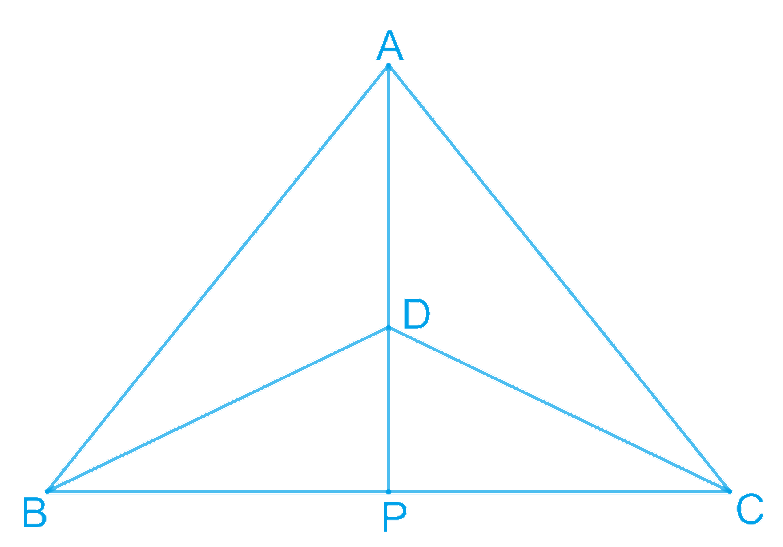Steps:

(i) In $$\Delta ABD$$ and $$\Delta ACD, \; AB = AC$$ (Given)

\begin{align}&BD = CD \text{(Given)}\\&AD = AD \text{(Common)}\\&\Delta ABD \cong \Delta ACD \\&\text{(By SSS congruence rule )}\end{align}

$\angle BAD = \angle CAD\;(\text{By}\;CPCT)\\ \angle BAP = \angle CAP \dots \ldots \ldots (1)$

(ii) In $$\Delta ABP$$ and $$\Delta ACP$$, $$AB = AC$$ (Given)

\begin{align} \angle BAP &= \angle CAP\;[\text{From equation (1)}] \\ AP &= AP\;(\text{Common})\\ \therefore \Delta ABP &\cong \Delta ACP\\text{By SAS}&\text { congruence rule})\end{align} \begin{align} \therefore BP = CP\;(\text{By CPCT}) \dots \dots (2)\end{align} (iii) From Equation (1), $\angle BAP = \angle CAP$ Hence, \(AP bisects $$\angle A$$.

In $$\Delta BDP$$ and $$\Delta CDP$$,

\begin{align}BD &= CD\;(\text{Given})\\DP &= DP\;(\text{Common})\\BP &= CP\\ [\text{From} &\text{ equation (2)}]\\ \\\therefore \Delta BDP &\cong \Delta CDP\\text{By SSS} &\text{ Congruence rule})\\\\ \therefore \Delta BDP &= \Delta CDP\\(\text{By} &\text{ CPCT})\ldots (3)\end{align} Hence, \(AP bisects $$\angle D$$.

(iv) $$\Delta BDP \cong \Delta CDP$$

\begin{align} \therefore 2\angle BPD &= \angle CPD\\text{By}\text{ CPCT}) &\ldots (4)\\ \angle BPD + \angle CPD &= 180^{\circ}\\(\text{Linear pair}& \text{ angles})\\\\ \angle BPD + \angle BPD &= 180^{\circ}\end{align} \(\angle BPD = 180^{\circ} [From Equation ($$2$$)]

$\angle BPD = 90^{\circ}\;\ldots \ldots \ldots (5)$

From Equations ($$2$$) and ($$5$$), it can be said that $$AP$$ is the perpendicular bisector of $$BC$$.

## Chapter 7 Ex.7.3 Question 2

$$AD$$ is an altitude of an isosceles triangle

$$ABC$$ in which $$AB = AC.$$ Show that

(i) $$AD$$ bisects $$BC$$

(ii) $$AD$$ bisects $$\angle A$$.

### Solution

What is Known?

AD is an altitude of an isosceles triangle $$ABC$$ in which $$AB\,\, AC.$$

To prove:

i) $$AD$$ bisects $$BC$$

ii) $$AD$$ bisects

Reasoning:

We can show triangle $$BAD$$ and $$CAD$$ congruent by using RHS congruency criterion and then we can say corresponding parts of congruent triangle are equal.

Steps: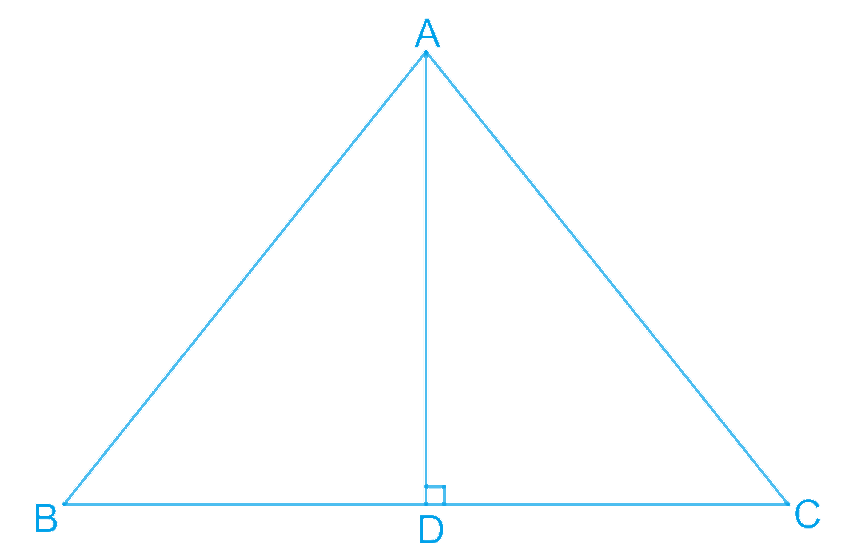(i) In $$\Delta BAD$$ and $$\Delta CAD$$,

\begin{align} \angle ADB &= \angle ADC\\text{Each }90^{\circ}&\text{ as }AD\text{ is the altitude})\\\\AB &= AC\;(\text{Given})\\AD &= AD\;(\text{Common})\\ \therefore \Delta BAD &\cong \Delta CAD\\(\text{By RHS }&\text{ Congruence rule})\end{align} \(\therefore BD = CD (By $$CPCT)$$

Hence, $$AD$$ bisects $$BC$$.

(ii) Also, by $$CPCT$$,

$$\angle BAD = \angle CAD$$

Hence, $$AD$$ bisects $$\angle A$$.

## Chapter 7 Ex.7.3 Question 3

Two sides $$AB$$ and $$BC$$ and median $$AM$$ of one triangle $$ABC$$ are respectively equal to sides $$PQ$$ and $$QR$$ and median $$PN$$ of $$\Delta PQR$$ (see the given figure). Show that:

(i) $$\Delta ABP \cong \Delta PQN$$

(ii) $$\Delta ABC \cong \Delta PQR$$

### Solution

What is Known?

Sides $$AB=PQ$$, $$BC=QR$$ and $$AM = PN$$ also $$AM$$ and $$PN$$ are medians.

To prove:

i) $$\Delta ABM\cong \Delta PQN$$

ii) $$\Delta ABC\cong \Delta PQR$$

Reasoning:

Using the median property we can show Triangles ABM and PQN are congruent by SSS congruency then we can say corresponding parts of congruent triangles are equal and then Triangles ABC and PQR by SAS congruency.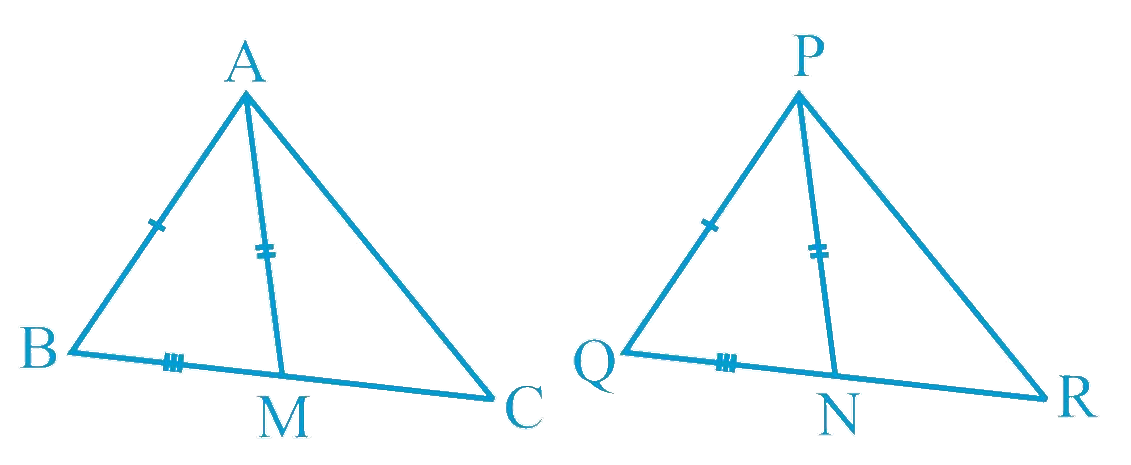Steps:

i) In $$\Delta ABC, AM$$ is the median to $$BC$$.

\begin{align} \therefore BM = \frac{1}{2} BC \end{align}

In $$\Delta PQR, PN$$ is the median to $$QR$$.

\begin{align} \therefore QN = \frac{1}{2} QR \end{align}

However, $$BC = QR$$ [Given]

\begin{align} & \therefore \frac{1}{2}BC = \frac{1}{2}QR\\ & \therefore BM = QN \ldots (1)\end{align}

In $$\Delta ABM$$ and $$\Delta PQN$$,

\begin{align}AB &= PQ\;(\text{Given})\\ BM &= QN\;\\&\text{[From Equation (1)}]\\ AM &= PN\;(\text{Given})\\ \Delta ABM &\cong \Delta PQN \\&\text{(By SSS congruence rule)} \end{align}

\begin{align}& \angle ABM = \angle PQN \;(\text{By }CPCT)\\ &\angle ABC = \angle PQR\dots (2) \end{align}

ii) In $$\Delta ABC$$ and $$\Delta PQR$$,

\begin{align}AB &= PQ\;(\text{Given})\\ \angle ABC &= \angle PQR \\&\text{[From Equation (2)}]\\\\BC &= QR\;(\text{Given})\\ \Delta ABC &\cong \Delta PQR \\&(\text{By SAS congruence rule})\end{align}

## Chapter 7 Ex.7.3 Question 4

$$BE$$ and $$CF$$ are two equal altitudes of a triangle $$ABC$$. Using RHS congruence rule, prove that the triangle $$ABC$$ is isosceles.

### Solution

What is Known?

$$BE$$ and $$CF$$ are two equal altitudes of a triangle $$ABC.$$

To prove:

Triangle $$ABC$$ is isosceles by using RHS congruence rule.

Reasoning:

We can show triangles $$BEC$$ and $$CFB$$ congruent by using RHS congruency and then we can say corresponding parts of congruent triangles are equal to prove the required result.

Steps: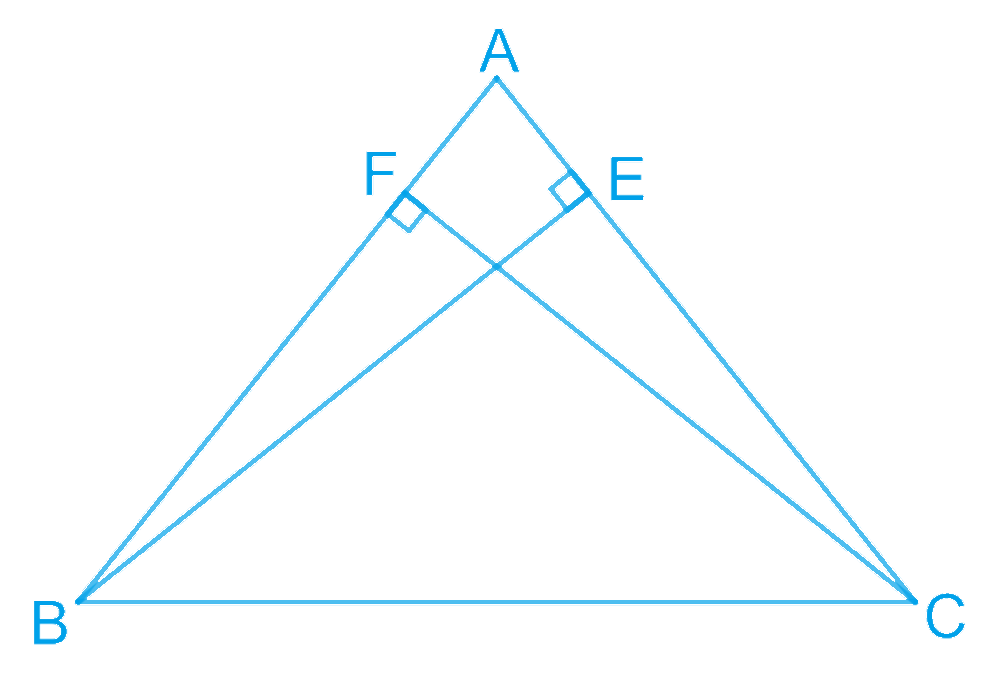In $$\Delta BEC$$ and $$\Delta CFB$$,

\begin{align} \angle BEC & = \angle CFB\;(\text{Each }90^{\circ})\\ BC &= CB\;(\text{Common})\\ BE &= CF\;(\text{Given})\\ \therefore \Delta BEC &\cong \Delta CFB\\ &\text{(By RHS congruency)} \end{align}

$$\therefore \angle BCE = \angle CBF (\text{By }CPCT)$$

$$\therefore AB = AC$$ (Sides opposite to equal angles of a triangle are equal) Hence, $$\Delta ABC$$ is isosceles.

## Chapter 7 Ex.7.3 Question 5

$$ABC$$ is an isosceles triangle with

$$AB = AC$$. Draw $$AP ⊥ BC$$ to show that $$\angle B = \angle C$$.

### Solution

What is Known?

$$ABC$$ is an isosceles triangle with $$AB \;\;AC$$ and $$AP \;BC$$

To prove:

$\angle \text{B}=\angle \text{C}$

Reasoning:

We can show triangles $$APB$$ and $$APC$$ congruent by using RHS congruency and then we can say corresponding parts of congruent triangles are equal to show the required result.

Steps: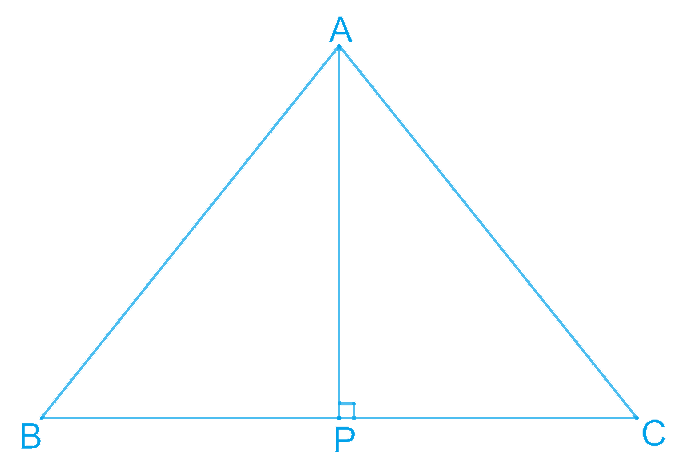In $$\Delta APB$$ and $$\Delta APC$$,

\begin{align} \angle APB & = \angle APC\;(\text{Each }90^{\circ})\\ AB &= AC \;(\text {Given})\\ AP& = AP \;(\text {Common})\\ \Delta APB &\cong \Delta APC \\&(\text {Using RHS congruence rule})\end{align}

$\angle B = \angle C\;(\text{By using }CPCT)$

Instant doubt clearing with Cuemath Advanced Math Program